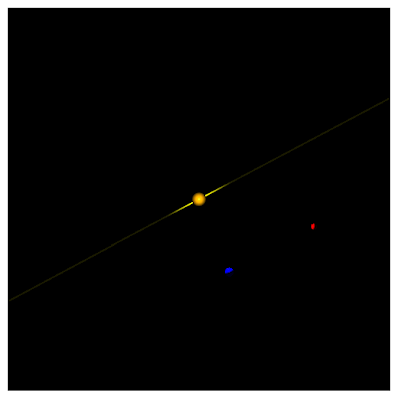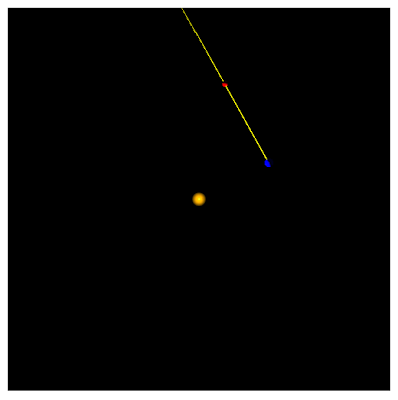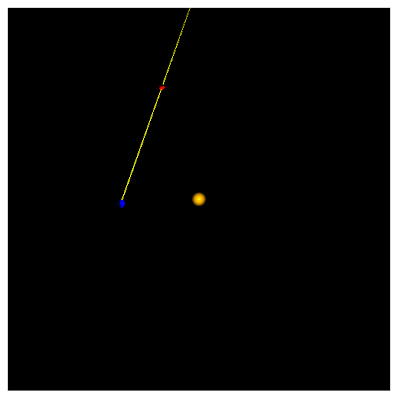## Saturday, June 23, 2012

‹prev | My Chain | next›

Before Galileo and the telescope, there were still motions in the heavens that baffled ancient astronomers. These were not dumb people by any stretch of the imagination, rather they were limited in their worldly (heavenly?) view. In other words, they lacked Gladius and CubicVR.js.

One of the things that defied explanation was the apparent retrograde motion of the outer planets. As planets revolve about the Sun over the course of months and years, they move in the sky. Over the course of time, they move eastward relative to the stars. But, every now and then, they switch direction and move westward for a few weeks or so. This was really hard to explain with the Earth at the center of the universe, but is easy to visualize with the Earth and other planets revolving around the Sun.

I already have Mars and the Earth revolving around the Sun. To visualize retrograde motion, I need to draw the line-of-sight from the Earth to Mars and out into space. I am using a very thin cylinder as the line. Now I need to figure out how to draw the line in Gladius.

First, I need to where both Mars and Earth are. I cannot simply ask for the X-Y coordinates of both planets, because their coordinates are fixed, but their frames of references are rotated. So I start by determining the amount of rotation of each:
``````      var marsRevolution = new engine.math.Vector3(
space.
findNamed( "mars-center-of-mass" ).
findComponent( "Transform" ).
rotation
);

var earthRevolution = new engine.math.Vector3(
space.
findNamed( "earth-center-of-mass" ).
findComponent( "Transform" ).
rotation
);``````
With the angles of rotation, I can get the X-Y location with simple trigonometry. The difference between Mars' and Earth's coordinates is then easy to calculate:
``````      var earthX = Math.cos(earthRevolution) * earthDistance
, earthY = Math.sin(earthRevolution) * earthDistance
, marsX =  Math.cos(marsRevolution) * marsDistance
, marsY = Math.sin(marsRevolution) * marsDistance``````
The difference in X-Y coordinates can then be used to determine the angle by which the line of sight needs to rotate thanks to the awesome power of `atan2()`:
``````
var y_diff = marsY - earthY
, x_diff = marsX - earthX
, angle = Math.atan2(x_diff, y_diff);``````
Finally, I rotate the line of sight marker by that angle:
``````      line_of_sight.
findComponent( "Transform" ).
setRotation( [0,0,-angle] );``````
The result is:The angle is correct, but the line goes through the Sun instead of starting from the Earth and running through Mars. First, I move the line-of-sight marker to the Earth:
``````      line_of_sight.
findComponent( "Transform" ).
setPosition( [earthX, earthY, 20] );``````
With that, the line of sight is now running through Mars and the Earth:To shift it so that it starts on the Earth and runs through Mars, I need a bit more trig (the cylinder is 50 units long, so I shift it by half):
``````      line_of_sight.
findComponent( "Transform" ).
setPosition( [earthX + 25*Math.sin(angle), earthY + 25*Math.cos(angle), 20] );
``````
And now, I can finally witness retrograde motion. Mars starts at a point in the sky that is to the right of the mid-point:As both Mars and the Earth revolve about the Sun, Mars's position in the sky continues to move to the left:But, as the Earth overtakes Mars, Mars slows:And then seems to travel backwards:Before resuming its leftward / eastward movement:That's pretty cool. When I first learned Java waaaay back in the day, I made a similar visualization. At the time I made an "applet" which is how old people used to make Flash visualizations before canvas. I think it may have been easier back then (I was certainly more proficient at trigonometry). But the visualization was limited to two dimensions. Now I have three dimensions available to me, which I will start exploring tomorrow.

Day #426